Like   Tweet
 /* styles */ June 25, 2020 Dear Readers, This is the tenth installment of DLPP’s “Expanding Your Horizons While Sheltering at Home.” The finalists of 2010 tell stories of people and countries struggling for identity through the clash of two cultures. Stories and essays of the contradictions within Nigerian/American diaspora are told by two gifted Nigerian writers, Adichie and Achebe. Ha Jin’s A Good Fall depicts the struggle of the Chinese/American diaspora; Zeitoun describes a Syrian American caught in the tragedy of Katrina; a complicated story of love and betrayal explores relationships of fathers and sons, lovers and brothers plays out in Cutting for Stone. The inhabitants of In The Valley of Mist in Kashmir, one of the most beautiful places on earth, are torn between Pakistan and India, the two countries vying for control of them. The young are often the most confused by the struggle for identity. In Book of Night Women, a young Jamaican slave of mixed heritage is lied to about who she is and pays the price; The Calligrapher’s Daughter shows another young woman’s fight for a brighter future during the Japanese rule of Korea; and in A Postcard from a Volcano, as in Wallace Steven’s poem by the same name, a young Prussian finds himself a stranger in the land of his birth. As desperate as these struggles for identity are, the quest for identity leads to acknowledging the truth and a personal sense of peace. Sometimes the world is so dark that it is difficult to see any hope but writers insist upon telling stories so that truth is revealed and tragedy can be avoided. War rarely comes darker than the description of the Bataan Death March researched in Tears in Darkness or in the conspiracy of countries to keep Africa hungry in Enough. These stories, too, provide a hope in that if we know the truth, we can work to ensure that such atrocities are not continued or repeated. The books of 2010 take us around the world and bring us home again, with a new understanding of ourselves, our fellow citizens, and our country. Stay safe. Be well. Read books.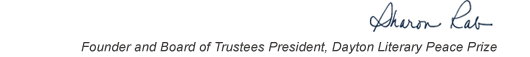table div table+table+table+table div table{width:100%;padding:0}table div table+table+table+table div table img{width:96.23%;padding:0;float:none}table div table+table+table+table div table td{width:100%;padding:0 1.88% 18px}/* styles */This list includes the winners and runners-up for 2010 and we have rich background information on each one on our website.

▪ Under Past Winners. scroll down to the year and click on it.
▪ If you click on the winners and runners-up, you will find the author’s photo and bio, a selection from the book, the judges’ citation, the writer’s reflection on literature and peace, and the option of watching the introduction and acceptance speech. If you click on the finalists, you will find a short description of each book.
 ▪ Under Past Winners. scroll down to the year and click on it.
 ▪ If you click on the winners and runners-up, you will find the author’s photo and bio, a selection from the book, the judges’ citation, the writer’s reflection on literature and peace, and the option of watching the introduction and acceptance speech. If you click on the finalists, you will find a short description of each book.

On the bottom right of the home page, you can click on COREScholar, which is built by the Chair of our Curriculum Committee Carol Loranger, Associate Dean of the College of Liberal Arts at Wright State, and maintained for us by Wright State University. There you can find interviews, TED Talks, articles, other books, films, etc. by and about each of our winning and runner-up authors. It is a great resource for students, book clubs, and readers who would like to explore an author in depth.

 table div table+table+table+table+table+table div table{width:100%;padding:0}table div table+table+table+table+table+table div table img{width:96.23%;padding:0;float:none}table div table+table+table+table+table+table div table td{width:100%;padding:0 1.88% 18px}/* styles *//* styles */ “Read global; buy local.” — Marlon James, DLPP 2009 Fiction Winner for The Book of Night Women
 table div table+table+table+table+table+table+table+table div table{width:100%;padding:0}table div table+table+table+table+table+table+table+table div table img{width:96.23%;padding:0;float:none}table div table+table+table+table+table+table+table+table div table td{width:100%;padding:0 1.88% 18px}/* styles */## 2010 Finalists - Fiction

 table div table+table+table+table+table+table+table+table+table+table div table{width:100%;padding:0}table div table+table+table+table+table+table+table+table+table+table div table img{width:96.23%;padding:0;float:none}table div table+table+table+table+table+table+table+table+table+table div table td{width:100%;padding:0 1.88% 18px}/* styles */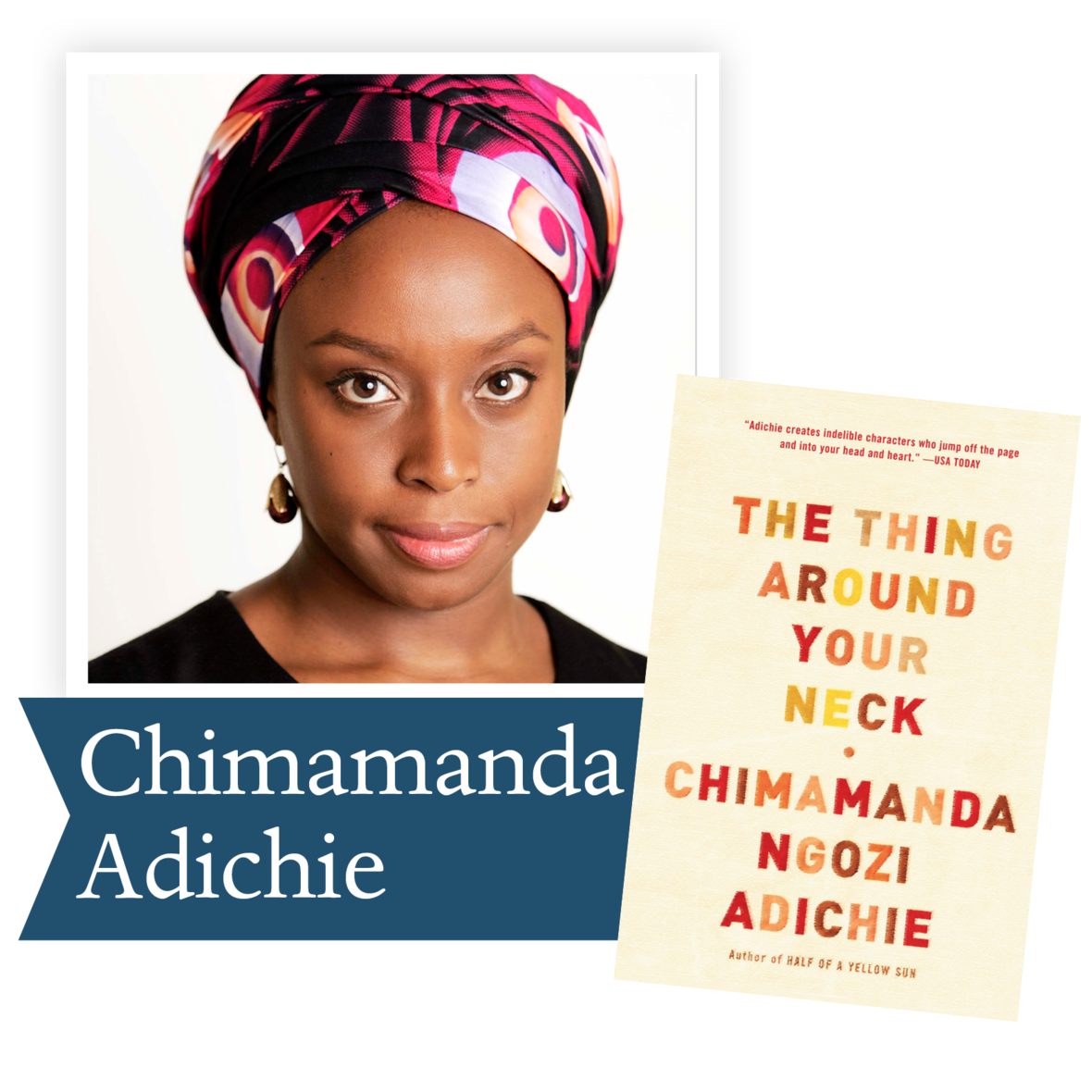The Thing Around Your Neck by Chimamanda Adiche (Knopf): Nigerian writer Chimamanda Ngozi Adichie turns her penetrating eye on both her native country and America in twelve dazzling stories that explore the collision of two cultures and the deeply human struggle to reconcile them.

 table div table+table+table+table+table+table+table+table+table+table+table+table div table{width:100%;padding:0}table div table+table+table+table+table+table+table+table+table+table+table+table div table img{width:96.23%;padding:0;float:none}table div table+table+table+table+table+table+table+table+table+table+table+table div table td{width:100%;padding:0 1.88% 18px}/* styles */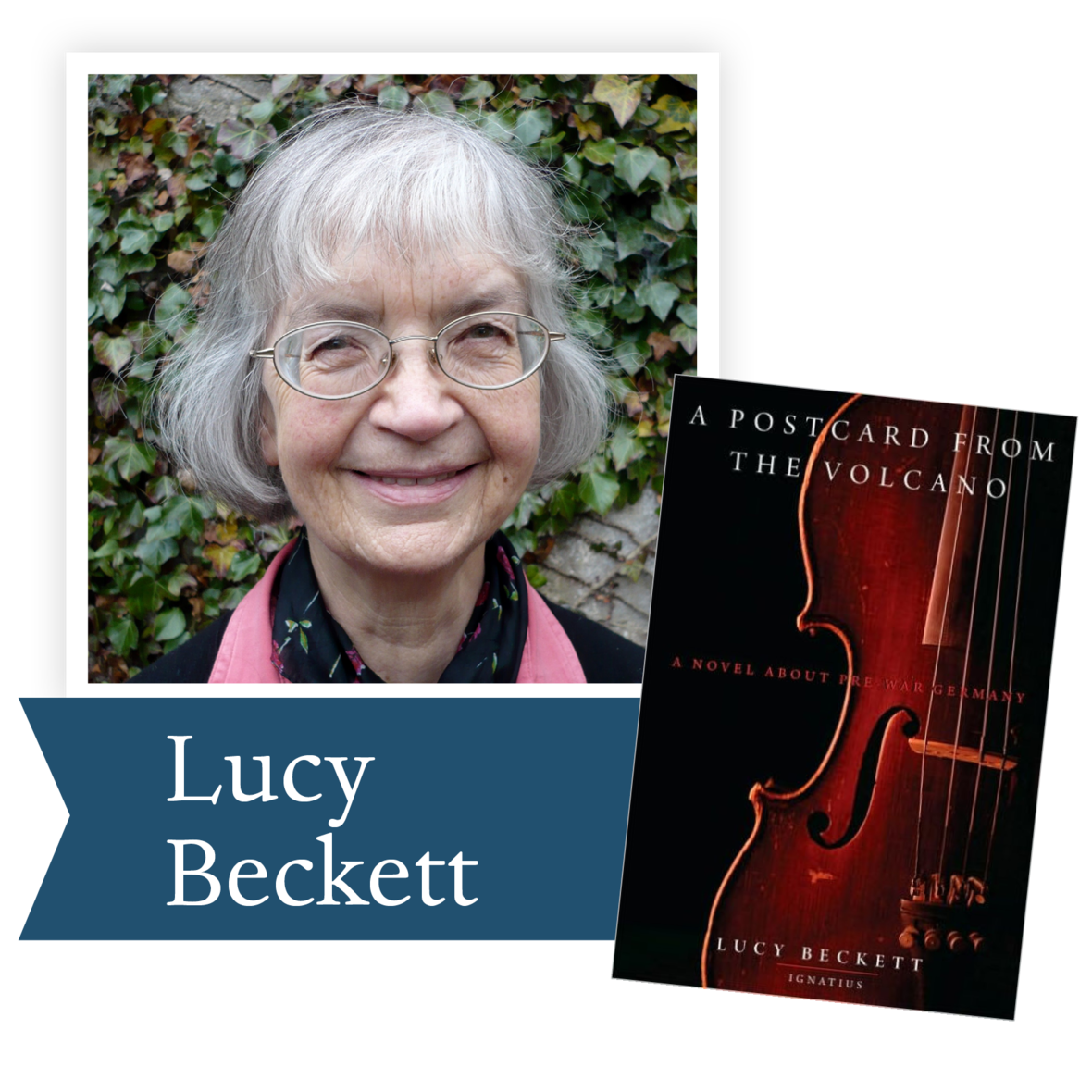A Postcard from the Volcano by Lucy Beckett (Ignatius Press): Beginning in 1914 and ending on the eve of World War II, this epic coming-of-age story follows a Prussian aristocrat as he confronts the ideologies that threaten the annihilation of millions of people.

 table div table+table+table+table+table+table+table+table+table+table+table+table+table+table div table{width:100%;padding:0}table div table+table+table+table+table+table+table+table+table+table+table+table+table+table div table img{width:96.23%;padding:0;float:none}table div table+table+table+table+table+table+table+table+table+table+table+table+table+table div table td{width:100%;padding:0 1.88% 18px}/* styles */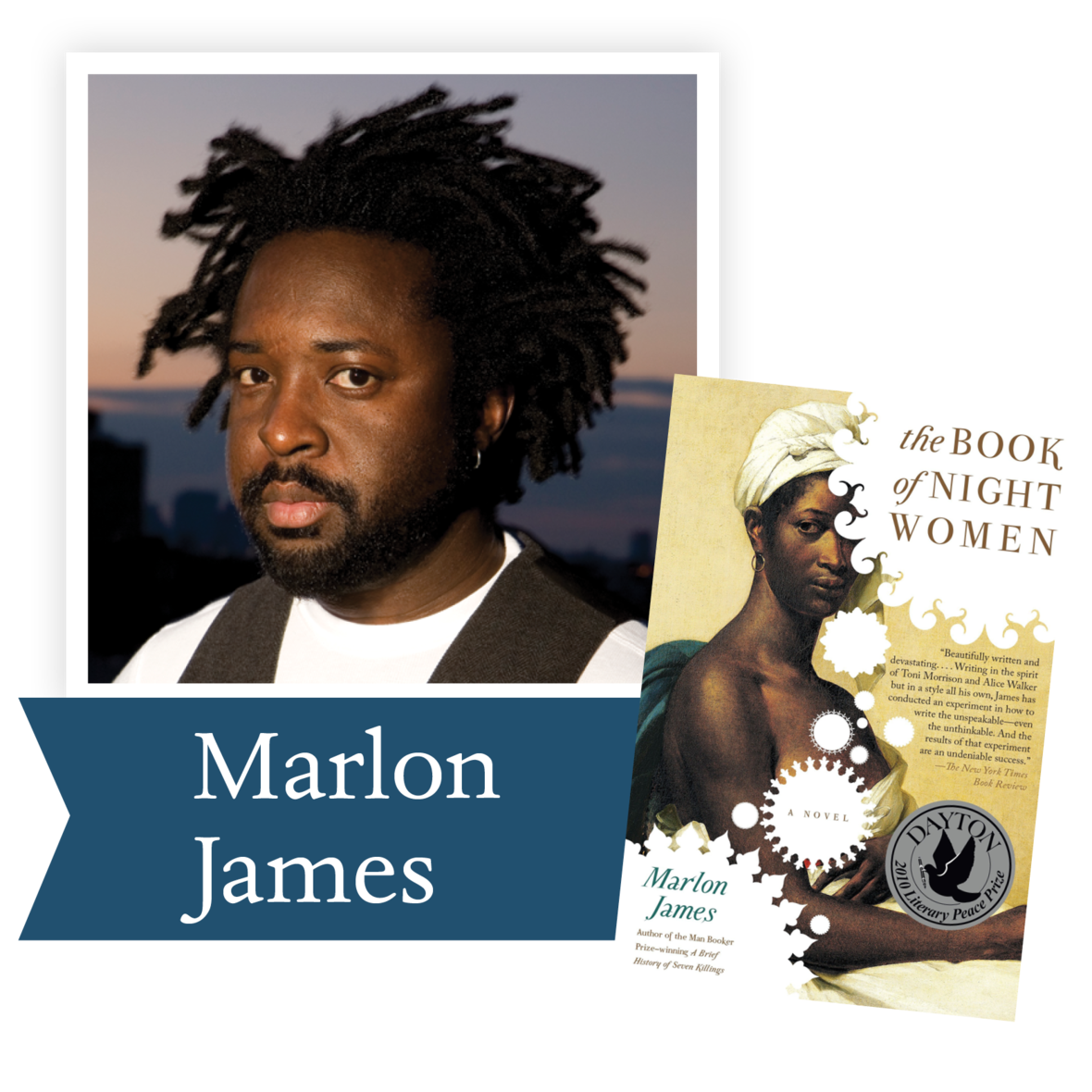The Book of Night Women by Marlon James (Penguin Group; G. P. Putham's Sons/ Riverhead Books): Born into slavery on a Jamaican sugar plantation at the end of the eighteenth century, a woman with dark, mysterious powers finds herself at the heart of a slave revolt plotted by the women around her.

 table div table+table+table+table+table+table+table+table+table+table+table+table+table+table+table+table div table{width:100%;padding:0}table div table+table+table+table+table+table+table+table+table+table+table+table+table+table+table+table div table img{width:96.23%;padding:0;float:none}table div table+table+table+table+table+table+table+table+table+table+table+table+table+table+table+table div table td{width:100%;padding:0 1.88% 18px}/* styles */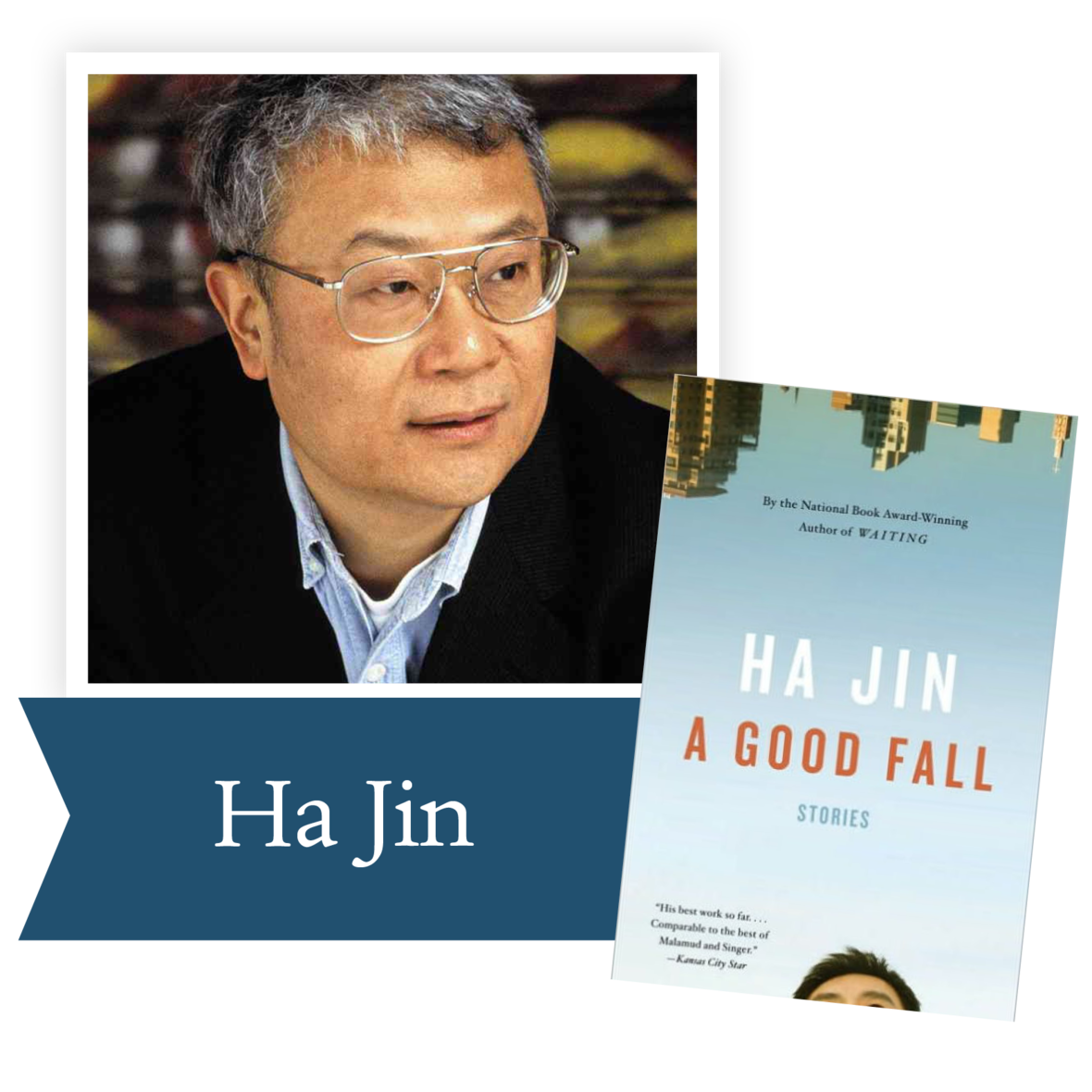A Good Fall by Ha Jin (Pantheon Books):
In this stark and insightful collection, acclaimed writer Ha Jin depicts the struggle of Chinese immigrants in America to remain loyal to their traditions as they explore the freedom that life in a new country offers.

 table div table+table+table+table+table+table+table+table+table+table+table+table+table+table+table+table+table+table div table{width:100%;padding:0}table div table+table+table+table+table+table+table+table+table+table+table+table+table+table+table+table+table+table div table img{width:96.23%;padding:0;float:none}table div table+table+table+table+table+table+table+table+table+table+table+table+table+table+table+table+table+table div table td{width:100%;padding:0 1.88% 18px}/* styles */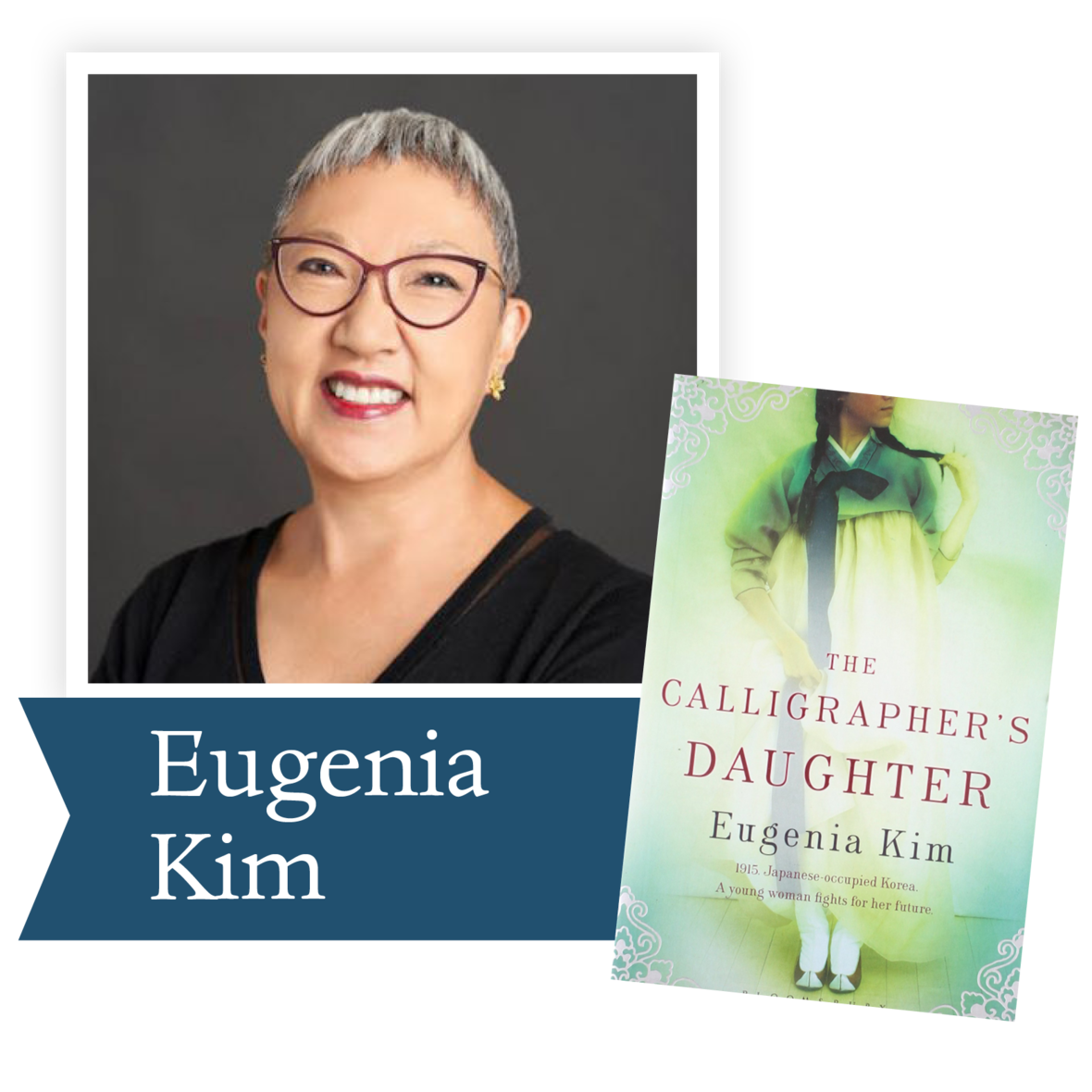The Calligrapher's Daughter by Eugenia Kim (Henry Holt and Company): In early-twentieth-century Korea, the privileged daughter of a calligrapher struggles to choose her own destiny while her country crumbles under Japanese occupation.

 table div table+table+table+table+table+table+table+table+table+table+table+table+table+table+table+table+table+table+table+table div table{width:100%;padding:0}table div table+table+table+table+table+table+table+table+table+table+table+table+table+table+table+table+table+table+table+table div table img{width:96.23%;padding:0;float:none}table div table+table+table+table+table+table+table+table+table+table+table+table+table+table+table+table+table+table+table+table div table td{width:100%;padding:0 1.88% 18px}/* styles */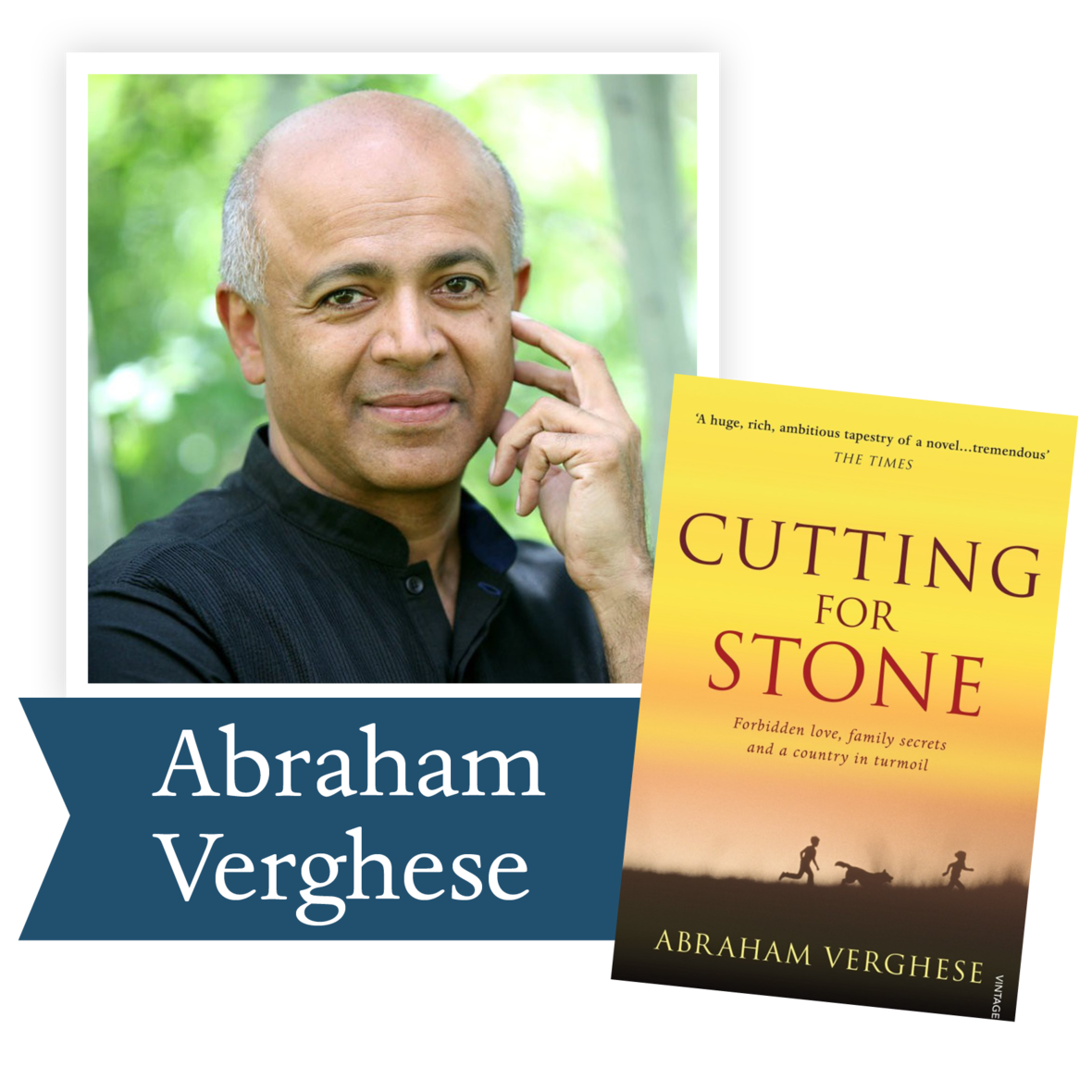Cutting for Stone by Abraham Verghese (Knopf):
A young Ethiopian doctor is forced to flee revolution in his homeland for New York City in this enthralling family saga of Africa and America, doctors and patients, exile and home.

 table div table+table+table+table+table+table+table+table+table+table+table+table+table+table+table+table+table+table+table+table+table+table div table{width:100%;padding:0}table div table+table+table+table+table+table+table+table+table+table+table+table+table+table+table+table+table+table+table+table+table+table div table img{width:96.23%;padding:0;float:none}table div table+table+table+table+table+table+table+table+table+table+table+table+table+table+table+table+table+table+table+table+table+table div table td{width:100%;padding:0 1.88% 18px}/* styles */## 2010 Finalists - Nonfiction

 table div table+table+table+table+table+table+table+table+table+table+table+table+table+table+table+table+table+table+table+table+table+table+table+table div table{width:100%;padding:0}table div table+table+table+table+table+table+table+table+table+table+table+table+table+table+table+table+table+table+table+table+table+table+table+table div table img{width:96.23%;padding:0;float:none}table div table+table+table+table+table+table+table+table+table+table+table+table+table+table+table+table+table+table+table+table+table+table+table+table div table td{width:100%;padding:0 1.88% 18px}/* styles */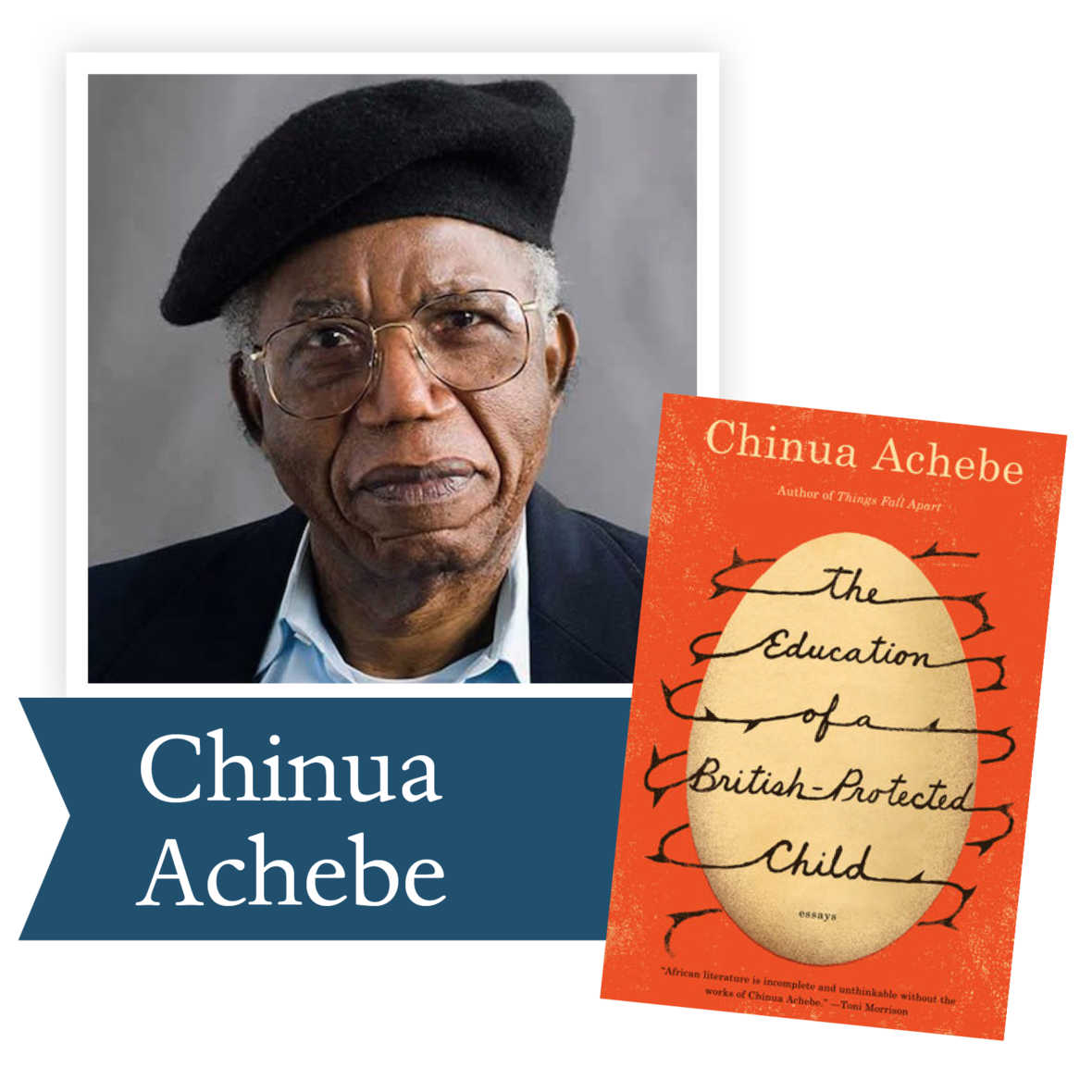The Education of a British-Protected Child by Chinua Achebe (Knopf): From the celebrated author of Things Fall Apart, a new collection of autobiographical essays—his first new book in more than twenty years.

 table div table+table+table+table+table+table+table+table+table+table+table+table+table+table+table+table+table+table+table+table+table+table+table+table+table+table div table{width:100%;padding:0}table div table+table+table+table+table+table+table+table+table+table+table+table+table+table+table+table+table+table+table+table+table+table+table+table+table+table div table img{width:96.23%;padding:0;float:none}table div table+table+table+table+table+table+table+table+table+table+table+table+table+table+table+table+table+table+table+table+table+table+table+table+table+table div table td{width:100%;padding:0 1.88% 18px}/* styles */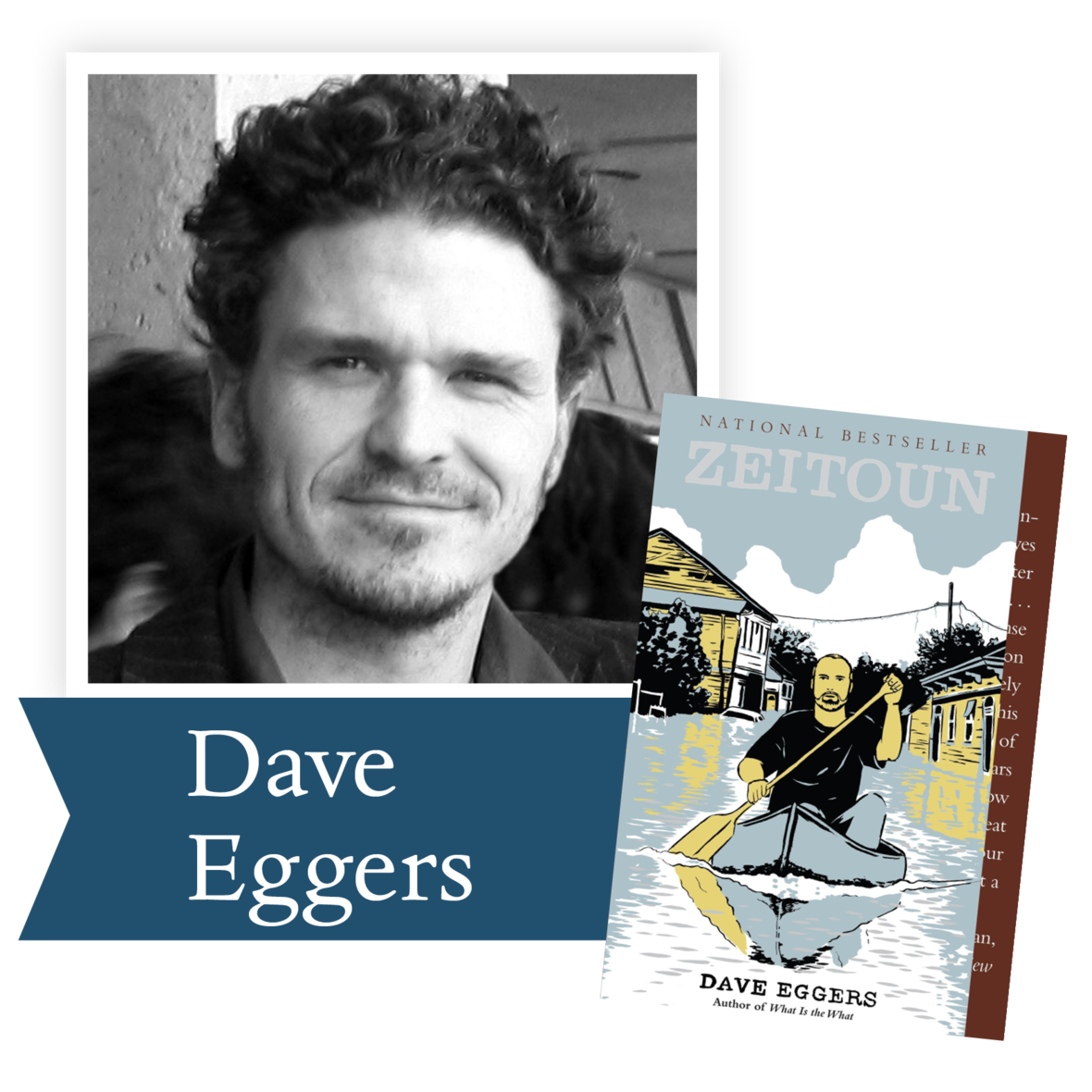Zeitoun by Dave Eggers (McSweeney's):
The meticulously researched story of a prosperous Syrian-American and father of four who chose to stay in New Orleans through Hurricane Katrina and protect his house and business—but then abruptly disappeared.

 table div table+table+table+table+table+table+table+table+table+table+table+table+table+table+table+table+table+table+table+table+table+table+table+table+table+table+table+table div table{width:100%;padding:0}table div table+table+table+table+table+table+table+table+table+table+table+table+table+table+table+table+table+table+table+table+table+table+table+table+table+table+table+table div table img{width:96.23%;padding:0;float:none}table div table+table+table+table+table+table+table+table+table+table+table+table+table+table+table+table+table+table+table+table+table+table+table+table+table+table+table+table div table td{width:100%;padding:0 1.88% 18px}/* styles */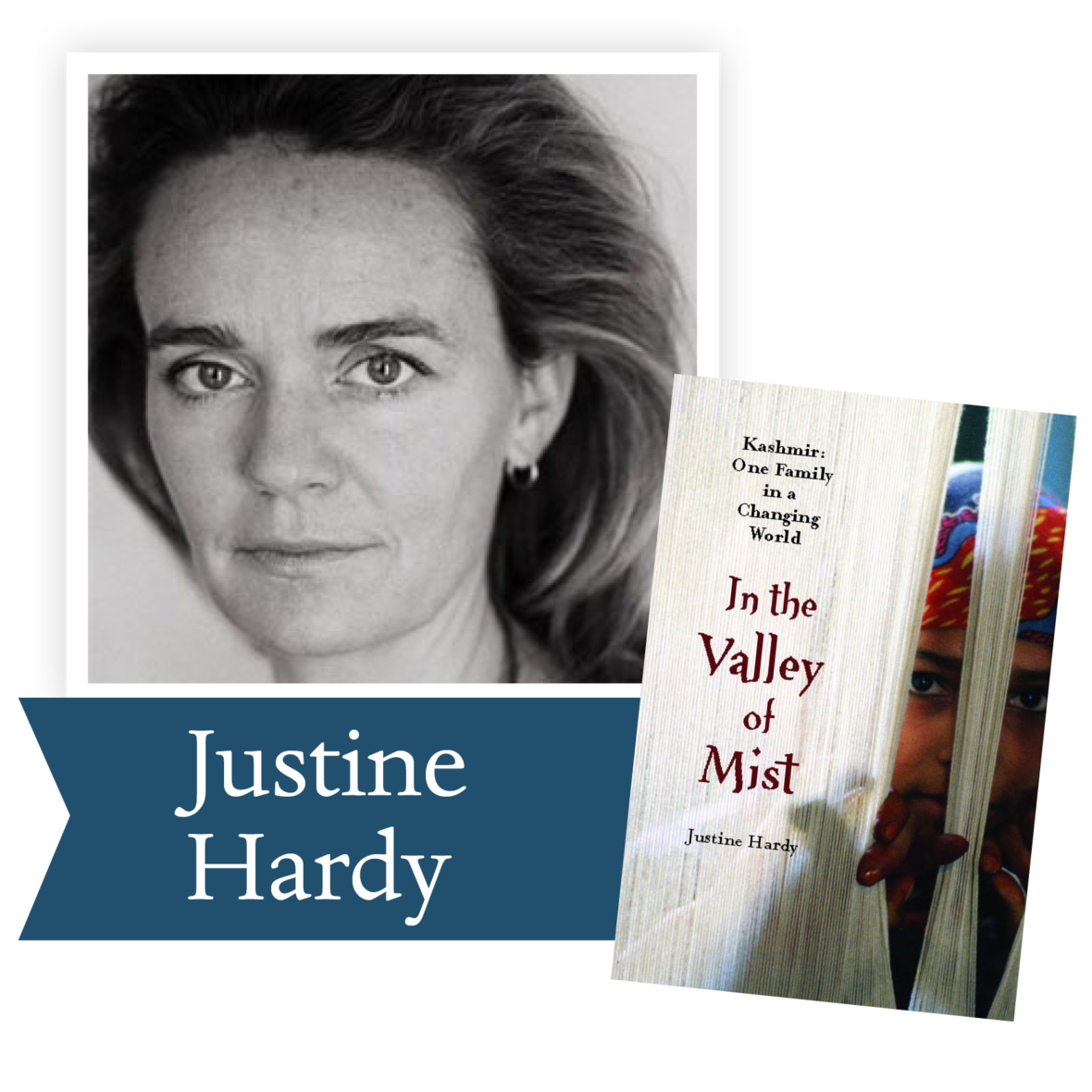In the Valley of Mist by Justine Hardy (Free Press): A personal, moving, and vibrant picture of the Kashmir Valley, one of the most beautiful and troubled places in the world—described through the experiences of one family, whose fortunes have changed dramatically with those of the region.

 table div table+table+table+table+table+table+table+table+table+table+table+table+table+table+table+table+table+table+table+table+table+table+table+table+table+table+table+table+table+table div table{width:100%;padding:0}table div table+table+table+table+table+table+table+table+table+table+table+table+table+table+table+table+table+table+table+table+table+table+table+table+table+table+table+table+table+table div table img{width:96.23%;padding:0;float:none}table div table+table+table+table+table+table+table+table+table+table+table+table+table+table+table+table+table+table+table+table+table+table+table+table+table+table+table+table+table+table div table td{width:100%;padding:0 1.88% 18px}/* styles */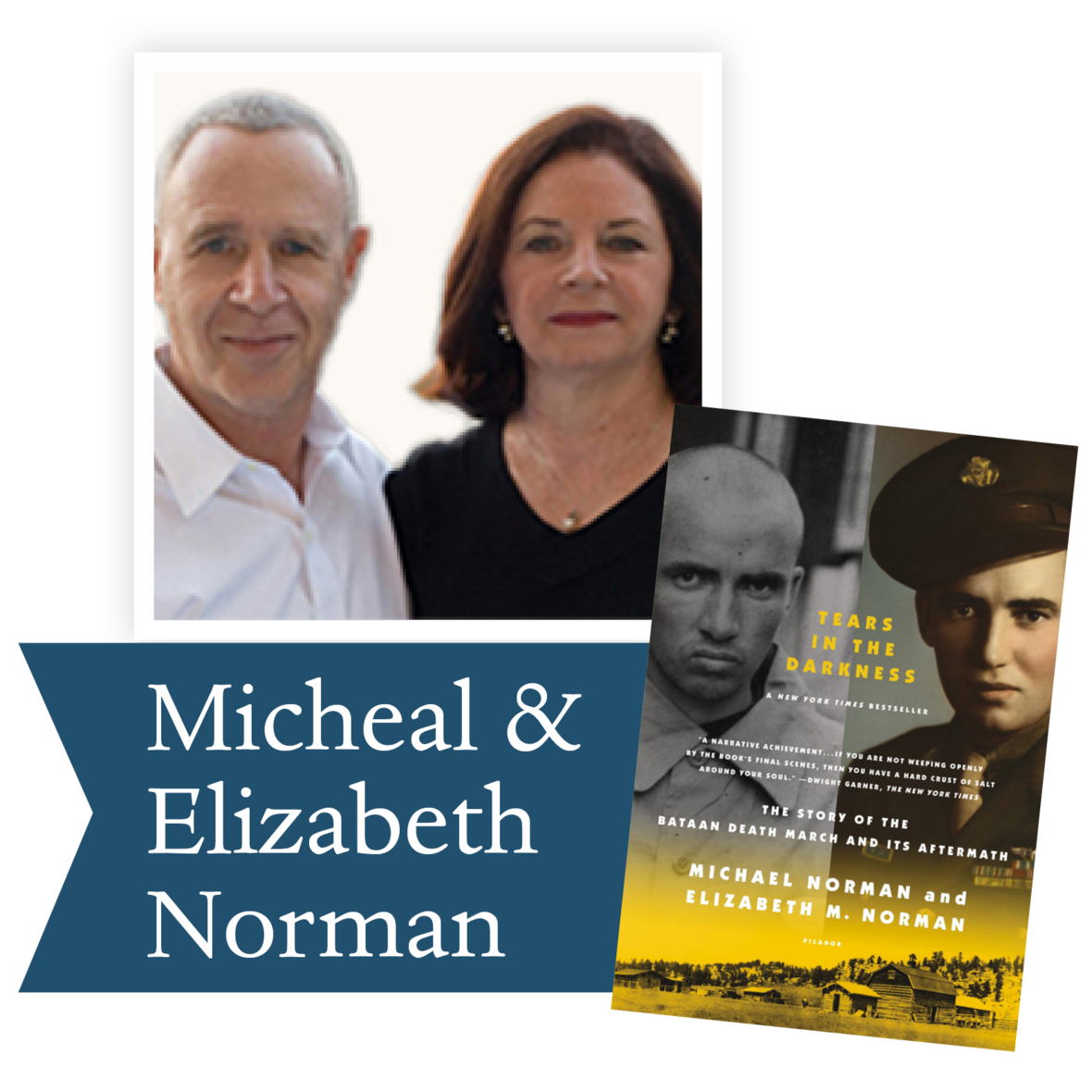Tears in the Darkness by Michael and Elizabeth Norman (Farrar, Straus and Giroux): Using the perspective of a young American soldier, this account of World War II’s Bataan Death March exposes the myths of war and shows the extent of suffering and loss on both sides.

 table div table+table+table+table+table+table+table+table+table+table+table+table+table+table+table+table+table+table+table+table+table+table+table+table+table+table+table+table+table+table+table+table div table{width:100%;padding:0}table div table+table+table+table+table+table+table+table+table+table+table+table+table+table+table+table+table+table+table+table+table+table+table+table+table+table+table+table+table+table+table+table div table img{width:96.23%;padding:0;float:none}table div table+table+table+table+table+table+table+table+table+table+table+table+table+table+table+table+table+table+table+table+table+table+table+table+table+table+table+table+table+table+table+table div table td{width:100%;padding:0 1.88% 18px}/* styles */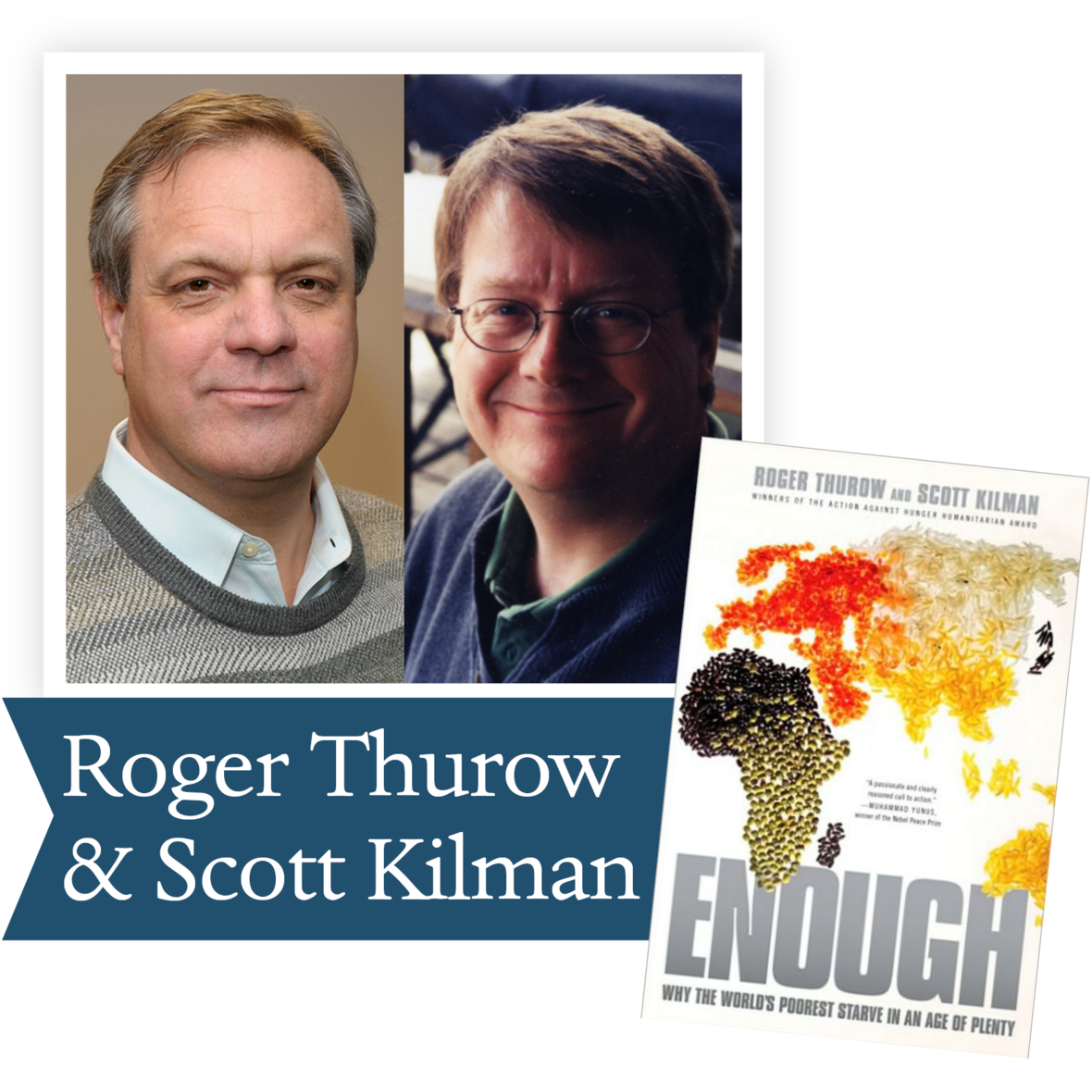Enough: Why the World's Poorest Starve in an Age of Plenty by Roger Thurow and Scott Kilman (Public Affairs): This powerful investigative narrative shows exactly how, in the past few decades, American, British, and European policies have conspired to keep Africa hungry and unable to feed itself.

 table div table+table+table+table+table+table+table+table+table+table+table+table+table+table+table+table+table+table+table+table+table+table+table+table+table+table+table+table+table+table+table+table+table+table div table{width:100%;padding:0}table div table+table+table+table+table+table+table+table+table+table+table+table+table+table+table+table+table+table+table+table+table+table+table+table+table+table+table+table+table+table+table+table+table+table div table img{width:96.23%;padding:0;float:none}table div table+table+table+table+table+table+table+table+table+table+table+table+table+table+table+table+table+table+table+table+table+table+table+table+table+table+table+table+table+table+table+table+table+table div table td{width:100%;padding:0 1.88% 18px}/* styles */## 2010 Ambassador Richard C. Holbrooke Distinguished Achievement Award WinnerGeraldine Brooks, Australian-born journalist, essayist, gives us a rich collection of literature to travel through time and distance. While today we are featuring the book that many consider to be her masterpiece, People of the Book, DLPP virtual book club recently read and discussed Year of Wonders about the plague in England. In her newest book, The Secret Chord, she depicts King David in all his humanity. You might also enjoy Caleb’s Crossing about the relationship of a young pre-revolutionary woman and her relationship with Caleb, a Native American who becomes her friend. And, of course, the Pulitzer Prize-winning March, where she builds an entire story from one line of Alcott’s Little Women to tell the story of the March father. You can’t go wrong with Geraldine Brooks.

This review of People of the Book from The Guardian will give you a taste of the power of this novel.

 table div table+table+table+table+table+table+table+table+table+table+table+table+table+table+table+table+table+table+table+table+table+table+table+table+table+table+table+table+table+table+table+table+table+table+table+table+table div table{width:100%;padding:0}table div table+table+table+table+table+table+table+table+table+table+table+table+table+table+table+table+table+table+table+table+table+table+table+table+table+table+table+table+table+table+table+table+table+table+table+table+table div table img{width:96.23%;padding:0;float:none}table div table+table+table+table+table+table+table+table+table+table+table+table+table+table+table+table+table+table+table+table+table+table+table+table+table+table+table+table+table+table+table+table+table+table+table+table+table div table td{width:100%;padding:0 1.88% 18px}/* styles */table div table+table+table+table+table+table+table+table+table+table+table+table+table+table+table+table+table+table+table+table+table+table+table+table+table+table+table+table+table+table+table+table+table+table+table+table+table+table+table div table{width:100%;padding:0}table div table+table+table+table+table+table+table+table+table+table+table+table+table+table+table+table+table+table+table+table+table+table+table+table+table+table+table+table+table+table+table+table+table+table+table+table+table+table+table div table img{width:96.23%;padding:0;float:none}table div table+table+table+table+table+table+table+table+table+table+table+table+table+table+table+table+table+table+table+table+table+table+table+table+table+table+table+table+table+table+table+table+table+table+table+table+table+table+table div table td{width:100%;padding:0 1.88% 18px}/* styles */Jesmyn Ward, a three-time finalist for the Dayton Literary Peace Prize, won the National Book Award for Sing, Unburied, Sing. Her work can educate us about the racial issues facing America today. Please join us in July to discuss this important book.

“The heart of Jesmyn Ward’s Sing, Unburied, Sing is story—the yearning for a narrative to help us understand ourselves, the pain of the gaps we’ll never fill, the truths that are failed by words and must be translated through ritual and song...Ward’s writing throbs with life, grief, and love, and this book is the kind that makes you ache to return to it.” —Buzzfeed

Ron Rollins, recently retired Dayton Daily News Ideas and Voices Editor, will moderate the discussion.

 table div table+table+table+table+table+table+table+table+table+table+table+table+table+table+table+table+table+table+table+table+table+table+table+table+table+table+table+table+table+table+table+table+table+table+table+table+table+table+table+table+table div table{width:100%;padding:0}table div table+table+table+table+table+table+table+table+table+table+table+table+table+table+table+table+table+table+table+table+table+table+table+table+table+table+table+table+table+table+table+table+table+table+table+table+table+table+table+table+table div table img{width:96.23%;padding:0;float:none}table div table+table+table+table+table+table+table+table+table+table+table+table+table+table+table+table+table+table+table+table+table+table+table+table+table+table+table+table+table+table+table+table+table+table+table+table+table+table+table+table+table div table td{width:100%;padding:0 1.88% 18px}/* styles */